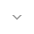belongs to Maven artifact com.android.support:support-compat:28.0.0-alpha1

# ObjectsCompat

``` public class ObjectsCompat ```
``` extends Object ``` ``` ```

 java.lang.Object ↳ android.support.v4.util.ObjectsCompat

This class consists of static utility methods for operating on objects.

## Summary

### Public methods

``` static boolean``` ``` equals(Object a, Object b) ```

Returns `true` if the arguments are equal to each other and `false` otherwise.

``` static int``` ``` hash(Object... values) ```

Generates a hash code for a sequence of input values.

``` static int``` ``` hashCode(Object o) ```

Returns the hash code of a non-`null` argument and 0 for a `null` argument.

### Inherited methodsFrom class ``` java.lang.Object ```

## Public methods

### equals

```boolean equals (Object a,
Object b)```

Returns `true` if the arguments are equal to each other and `false` otherwise.

Consequently, if both arguments are `null`, `true` is returned and if exactly one argument is `null`, `false` is returned. Otherwise, equality is determined by using the `equals` method of the first argument.

Parameters
`a` `Object`: an object

`b` `Object`: an object to be compared with `a` for equality

Returns
`boolean` `true` if the arguments are equal to each other and `false` otherwise

• `equals(Object)`

### hash

`int hash (Object... values)`

Generates a hash code for a sequence of input values. The hash code is generated as if all the input values were placed into an array, and that array were hashed by calling `hashCode(Object[])`.

This method is useful for implementing `hashCode()` on objects containing multiple fields. For example, if an object that has three fields, `x`, `y`, and `z`, one could write:

``` @Override public int hashCode() {
return ObjectsCompat.hash(x, y, z);
}
```
Warning: When a single object reference is supplied, the returned value does not equal the hash code of that object reference. This value can be computed by calling `hashCode(Object)`.

Parameters
`values` `Object`: the values to be hashed

Returns
`int` a hash value of the sequence of input values

• `hashCode(Object[])`
`int hashCode (Object o)`
Returns the hash code of a non-`null` argument and 0 for a `null` argument.
`o` `Object`: an object